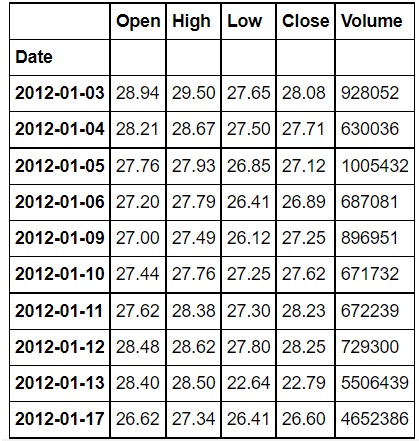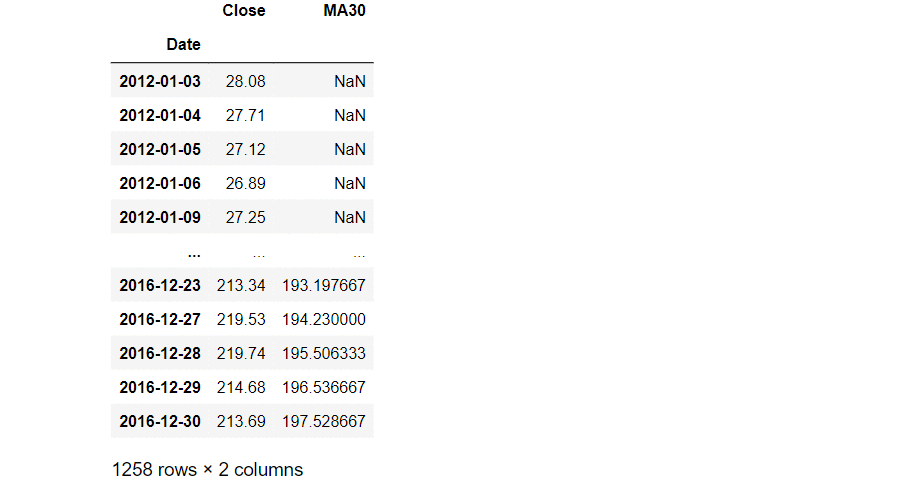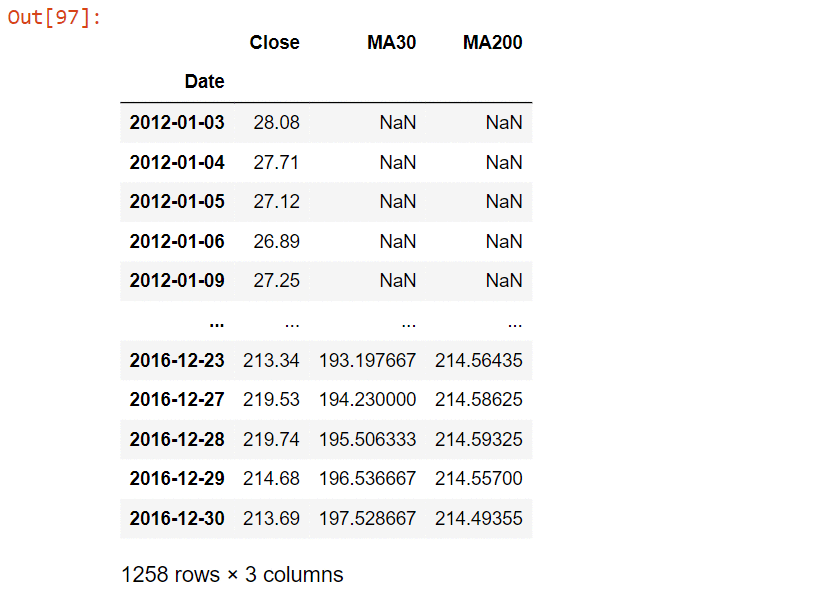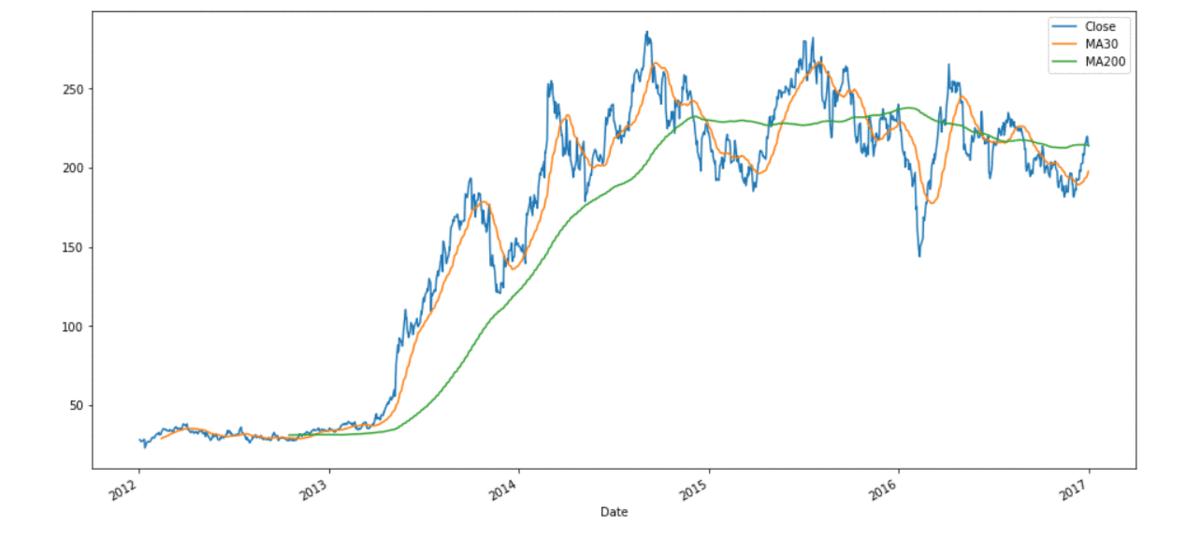Open in App
Not now

# Pandas – Rolling mean by time interval

• Difficulty Level : Hard
• Last Updated : 24 Oct, 2021

In this article, we will be looking at how to calculate the rolling mean of a dataframe by time interval using Pandas in Python.

Pandas dataframe.rolling() is a function that helps us to make calculations on a rolling window. In other words, we take a window of a fixed size and perform some mathematical calculations on it.

Syntax: DataFrame.rolling(window, min_periods=None, center=False, win_type=None, on=None, axis=0).mean()

Parameters :

• window : Size of the window. That is how many observations we have to take for the calculation of each window.
• min_periods : Least number of observations in a window required to have a value (otherwise result is NA).
• center : It is used to set the labels at the center of the window.
• win_type : It is used to set the window type.
• on : Datetime column of our dataframe on which we have to calculate rolling mean.
• axis : integer or string, default 0

Dataset Used: Tesla_Stock

## Python3

 `# import pandas as pd ` `import` `pandas as pd `

## Python3

 `# importing Data ` `tesla_df ``=` `pd.read_csv(``'Tesla_Stock.csv'``, index_col``=``'Date'``,  ` `                       ``parse_dates``=``True``) ` ` `  `# printing the dataFrame ` `tesla_df.head(``10``) `

Output:We will be calculating the rolling mean of the column ‘Close’ of the DataFrame.

## Python3

 `# Updating the dataFrame with just the  ` `# column 'Close' as others columns are  ` `# of no use right now we have used .to_frame ` `# which converts Series to a DataFrame. ` `tesla_df ``=` `tesla_df[``'Close'``].to_frame() ` ` `  ` `  `# calculating Rolling mean and storing it  ` `# into a new column of existing dataFrame ` `# we have set the window as 30 and rest all ` `# parameters are set to default. ` `tesla_df[``'MA30'``] ``=` `tesla_df[``'Close'``].rolling(``30``).mean() ` ` `  `# Rolling mean is also called as Moving Average , ` `# hence we have used the notation MA ` `# and MA30 is the moving average (rolling mean)  ` `# of 30 days ` ` `  `# printing dataframe ` `tesla_df`

Output:The First 29 rows of the column MA30 will have a value NULL  and the first non NULL value will be at row 30. Now we will be calculating the rolling mean with a window of 200.

## Python3

 `# calculating Rolling mean and storing it into ` `# a new column of existing dataFrame we have set ` `# the window as 200 and rest all parameters are  ` `# set to default. ` `tesla_df[``'MA200'``] ``=` `tesla_df[``'Close'``].rolling(``200``).mean() ` ` `  `# Rolling mean is also called as Moving Average, hence ` `# we have used the notation MA and MA200 is the moving ` `# average (rolling mean) of 200 days ` ` `  `# printing dataframe ` `tesla_df`

Output:For ‘MA200’ the first non-NULL will be at row 200. Now lets plot ‘MA30’ , ‘MA200’ and  ‘Close’ for better visualization

## Python3

 `# importing matplotlib module ` `import` `matplotlib.pyplot as plt ` `plt.style.use(``'default'``) ` ` `  `# %matplotlib inline: only draw static ` `# images in the notebook ` `%``matplotlib inline ` ` `  `tesla_df[[``'Close'``, ``'MA30'``, ``'MA200'``]].plot( ` `  ``label``=``'tesla'``, figsize``=``(``16``, ``8``)) `

OUTPUT:My Personal Notes arrow_drop_up
Related Articles